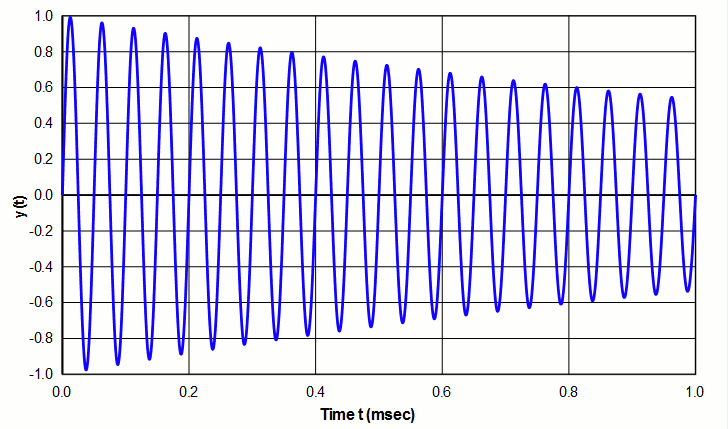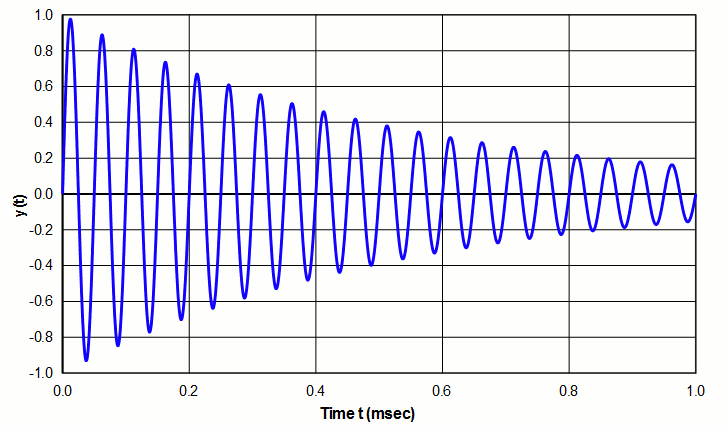# $$Q$$ (quality factor)

$$Q$$ (quality factor) is a property that relates the total stored energy to the energy dissipated (loss) —

\begin{align} \label{eq:13601a} Q = 2\pi \left[ \frac{\textsf{Energy stored}}{\textsf{Energy dissipated per cycle}} \right] \end{align}

The "energy dissipated per cycle" is the external energy (work) that would be needed in order to maintain a fixed amount of oscillation.

If the numerator and denominator are multiplied by the frequency $$f$$ (cycles/sec), the denominator becomes "Energy dissipated/sec" (i.e., Power), so the above equation can also be written as —

\begin{align} \label{eq:13602a} Q = 2\pi \, f \left[ \frac{\textsf{Energy stored}}{\textsf{Power}} \right] \end{align}

$$Q$$ has no units.

$$Q$$ can be determined for any system (electrical, mechanical, etc.) or any single component or portion of a system. Note that for a "perfect" system (i.e., no energy dissipation), $$Q$$ is infinite.

## Interpretation

### Resonant system

For a freely oscillating resonant system, $$Q$$ indicates the rate at which oscillation decays due to energy loss. A high $$Q$$ indicates relatively slow decay (example of figure 1) whereas a low $$Q$$ indicates relatively rapid decay (example of figure 2 - see log decrement ).Figure 1. System with high $$Q$$ (slow decay)Figure 2. System with low $$Q$$ (rapid decay)

In an ultrasonic system the energy loss may come from internal friction within a vibrating material (which will show up as a temperature rise), energy that is transferred to the support structure or air, or energy that is transferred to the load. In addition, a transducer will have electrical (resistive) losses due to current flow.

### Nonresonant system

Although Q is often applied to resonant systems, this is not a requirement. In fact, equations \eqref{eq:13601a} and \eqref{eq:13602a} can equally be applied to nonresonant systems — in particular, to capacitors and inductors.

A nonideal capacitor (i.e., a capacitor with loss) can be represented as an ideal capacitor $$C$$ in series with a resistor $$R_C$$.

For a capacitor —

\begin{align} \label{eq:13603a} Q &= \frac{R_C}{(1/2\pi \, f \, C)} \\[0.7em]%eqn_interline_spacing &= R \, (2\pi \, f \, C) \nonumber \end{align}

For an inductor —

\begin{align} \label{eq:13604a} Q &= \frac{R_L}{(2\pi \, f \, L)} \end{align}

where —

 $$R_C$$ = equivalent resistance of capacitor $$R_L$$ = equivalent resistance of inductor $$C$$ = capacitance $$L$$ = inductance $$f$$ = frequency

## Q for resonator materials

For materials used in ultrasonic resonators, the following are relevant.

1. $$Q$$ is a material property (in a similar manner to the modulus of elasticity). However, the test conditions must be specified because $$Q$$ may depend on:
1. The material's heat treatment. For example, D2 tool steel has significantly lower loss (power), and hence higher $$Q$$, when it is hardened.
2. The strain to which the part is subjected. Often $$Q$$ will decrease as the strain increases but this depends on the particular material.
2. $$Q$$ is generally independent of frequency.
3. For piezoelectric cermics $$Q$$ depends on the operating conditions (static compression, electrical field, temperature, etc.).

If $$Q$$ of a material is known then the theoretical power dissipated by a resonator can be calculated if the stored energy (either potential energy (strain energy) or kinetic energy) can be determined from equation \eqref{eq:13602a} —

\begin{align} \label{eq:13605a} \textsf{Power} = 2\pi \, f \left[ \frac{\textsf{Energy stored}}{Q} \right] \end{align}

### Data for materials

Neppiras (table 4, p. 146) cites the following $$Q$$ values.

Material $$Q$$
Naval brass 3000
Aluminum bronze 17000
K-monel 5300
Duralumin (DTD 363) 50000
Hiduminium (?) 100000
Tool steel (KE 672) 1400
Titanium (318A == Ti-6Al-4V) 24000

Wuchinich (1) (figure 10) measured the following values by striking a flexing "chime". However, the strains were low so the cited values may not be relevant at typical ultrasonic strains.

Material Condition $$Q$$
Ti-6Al-4V As received 2000 - 6000
Ti-6Al-4V Annealed 18000 - 22000
17-4PH stainless steel Annealed 7000
PH15-7Mo stainless Annealed 17000
PH15-7Mo stainless Hardened 17000
PH13-8Mo stainless Annealed 10000
Custom 455 stainless H900 10000
MACOR machineable ceramic As received 5000

## $$Q$$ measurement

For a resonant system $$Q$$ can be determined by —